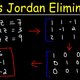• 300 BCE

Nine Chapters on the Mathematical Art

Authored in China and was the first place the elimination method appeared.
• 650

• Issac Newton

Issac Newton wrote notes which described the method and was the first to do so in Europe.
• Carl Friedrich Gauss

Gauss devised an algorithm to solve least-squares problems and referred to the method as "commonly known".
• Gauss-Seidel algorithm

A finer method which was used for his work on the triangulation of Hanover.
• Arthur Cayley

Matrices were introduced into mathematics
• Wilhelm Jordan

Jordan wrote a method for elimination for transforming a matrix into reduced echelon form
• B.-I. Clasen

B.-I. Clasen wrote a similar method as Jordan in the same year.
• Manchester Baby

The method of elimination was also implemented in the first stored-program digital computer.
• Current uses

The Guass-Jordan Elimination Method is now currently used in mathematics classes such as algebra 1, algebra 2, linear algebra, etc. to solve systems of linear equations.